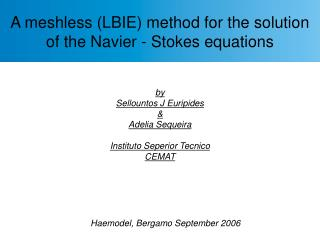# by Sellountos J Euripides & Adelia Sequeira Instituto Seperior Tecnico CEMAT - PowerPoint PPT PresentationDownload Presentationby Sellountos J Euripides & Adelia Sequeira Instituto Seperior Tecnico CEMAT

by Sellountos J Euripides & Adelia Sequeira Instituto Seperior Tecnico CEMATDownload Presentation## by Sellountos J Euripides & Adelia Sequeira Instituto Seperior Tecnico CEMAT

- - - - - - - - - - - - - - - - - - - - - - - - - - - E N D - - - - - - - - - - - - - - - - - - - - - - - - - - -
##### Presentation Transcript

1. A meshless (LBIE) method for the solution of the Navier - Stokes equations by Sellountos J Euripides & Adelia Sequeira Instituto Seperior Tecnico CEMAT Haemodel, Bergamo September 2006

2. Motivation of Meshless Methods: Easy to model • Meshing and remeshing of complex geometries relevant to blood flow problems (stenosed, curved or bifurcating vessels) is easy with the addition-movement of nodal points

3. Motivation of Meshless Methods: Easy to model • Meshing and remeshing of complex geometries relevant to blood flow problems (stenosed, curved or bifurcating vessels) is easy with the addition-movement of nodal points • Meshless methods: Computational method related to surface reconstruction techniques

4. Motivation of Meshless Methods: Easy to model • Meshing and remeshing of complex geometries relevant to blood flow problems (stenosed, curved or bifurcating vessels) is easy with the addition-movement of nodal points • Meshless methods: Computational method related to surface reconstruction techniques • Local solution of the boundary-domain integral equations

5. Motivation of Meshless Methods: Easy to model • Meshing and remeshing of complex geometries relevant to blood flow problems (stenosed, curved or bifurcating vessels) is easy with the addition-movement of nodal points • Meshless methods: Computational method related to surface reconstruction techniques • Local solution of the boundary-domain integral equations • Approximation of the unknown field with randomly distributed nodal points only • System of equations are in band form • In small vessels blood behaves as a shear thinning (and viscoelastic fluid)

6. Nodal Support, Connectivity and Interpolation Support domain of a nodal point Every nodal point has an associated circular region of influence

7. Nodal Support, Connectivity and Interpolation Neighborhood of a nodal point Support domain of a nodal point Every nodal point has an associated circular region of influence

8. Nodal Support, Connectivity and Interpolation Support domain of a nodal point Every nodal point has an associated circular region of influence Neighborhood of a nodal point Interpolation of unknown field

9. Generalized Navier – Stokes equations • Conservation of mass • Conservation of momentum • Shear stress • Vorticity • Strain rate tensor • Viscosity is assumed to be shear strain rate or shear stress dependant Armin Leuprecht and Karl Perktold

10. Generalized Navier – Stokes equations Velocity - vorticity scheme • The fluid motion scheme is partitioned to kinematics • and kinetics • decomposition of velocity and viscosity to a mean and a perturbed value

11. Generalized Navier – Stokes equations Velocity - vorticity scheme Kinematics Integral Representation Skerget and Hribersek

12. Generalized Navier – Stokes equations Velocity - vorticity scheme Kinetics Integral Representation

13. Generalized Navier – Stokes equations Velocity - vorticity scheme Kinematics Local Integral Representation Compation solution • Satisfies linear part of the differential operator • Equals to the fundamental on the local boundary

14. Generalized Navier – Stokes equations Velocity - vorticity scheme Kinetics Local Integral Representation

15. Meshless LBIE – Integral representations

16. Meshless LBIE – Integral representations

17. Meshless LBIE – Integral representations

18. Discretization and Numerical Evaluation of Integrals Involved Integrals

19. Discretization and Numerical Evaluation of Integrals • Arc integrals Involved Integrals

20. Discretization and Numerical Evaluation of Integrals • Boundary integrals Involved Integrals

21. Discretization and Numerical Evaluation of Integrals • Volume integrals Involved Integrals

22. Discretization and Solution Procedure

23. Discretization and Solution Procedure

24. Discretization and Solution Procedure Approximation of Boundary Vorticity ω Computation of shear rate and new nodal viscosity

25. Discretization and Solution Procedure

26. Discretization and Solution Procedure

27. Discretization and Solution Procedure Check vorticity’s convergence / Iteration decision

28. Newtonian Flow

29. Newtonian Flow

30. Newtonian Flow

31. Newtonian Flow

32. Newtonian Flow

33. Stenosis problem - qualitative example

34. Stenosis problem- qualitative example

35. Stenosis problem- qualitative example

36. Stenosis problem- qualitative example

37. Stenosis problem- qualitative example

38. Stenosis problem- qualitative example

39. Stenosis problem- qualitative example

40. Stenosis problem- qualitative example

41. Stenosis problem- qualitative example

42. Stenosis problem- qualitative example

43. Stenosis problem- qualitative example

44. Stenosis problem- qualitative example

45. Stenosis problem- qualitative example

46. Stenosis problem- qualitative example

47. Conclusions – Future work • Mesh free method, only points are needed for the interpolation • Solution of boundary integral equation • Use of other test functions instead of fundamental solution • Hypersingular integral equation for boundary points in kinematics equation Thanks for your attention